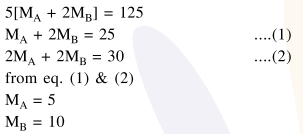# 5 moles of AB2 weigh

Question:

5 moles of $\mathrm{AB}_{2}$ weigh $125 \times 10^{-3} \mathrm{~kg}$ and 10 moles of $\mathrm{A}_{2} \mathrm{~B}_{2}$ weigh $300 \times 10^{-3} \mathrm{~kg}$. The molar mass of $\mathrm{A}\left(\mathrm{M}_{\mathrm{A}}\right)$ and molar mass of $\mathrm{B}\left(\mathrm{M}_{\mathrm{B}}\right)$ in $\mathrm{kg} \mathrm{mol}^{-1}$ are :

1. $\mathrm{M}_{\mathrm{A}}=50 \times 10^{-3}$ and $\mathrm{M}_{\mathrm{B}}=25 \times 10^{-3}$

2. $\mathrm{M}_{\mathrm{A}}=25 \times 10^{-3}$ and $\mathrm{M}_{\mathrm{B}}=50 \times 10^{-3}$

3. $\mathrm{M}_{\mathrm{A}}=5 \times 10^{-3}$ and $\mathrm{M}_{\mathrm{B}}=10 \times 10^{-3}$

4. $\mathrm{M}_{\mathrm{A}}=10 \times 10^{-3}$ and $\mathrm{M}_{\mathrm{B}}=5 \times 10^{-3}$

Correct Option: , 3

Solution: